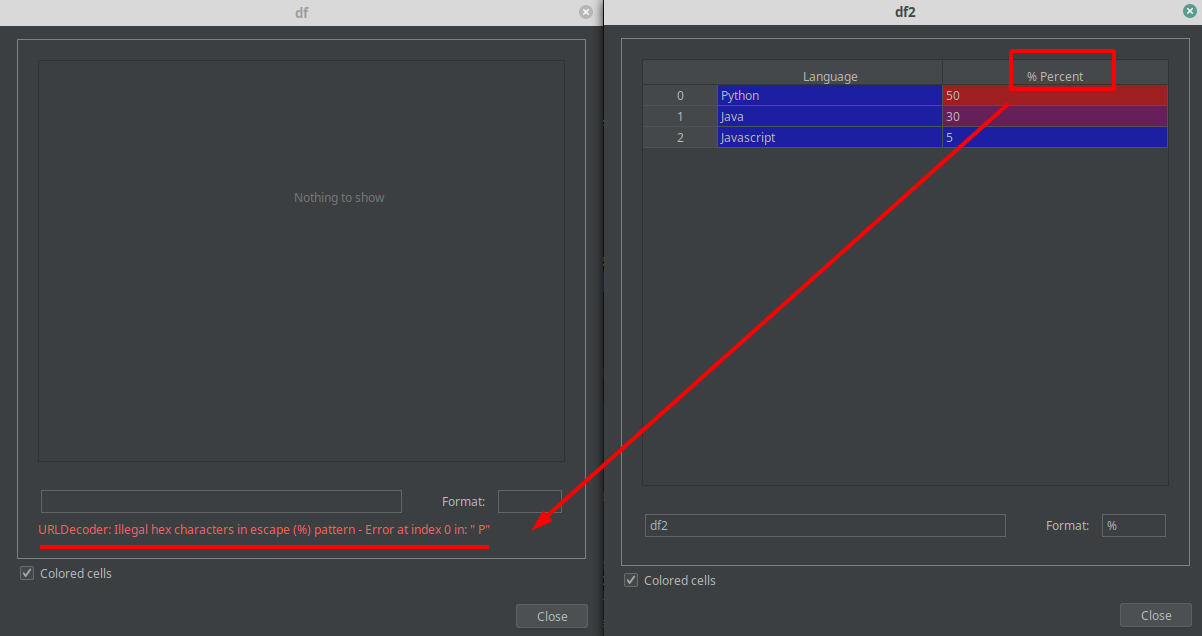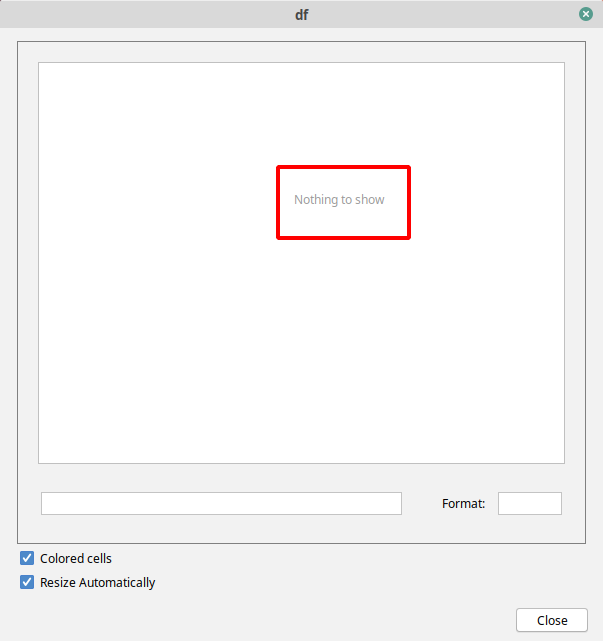Have you tried to view a Pandas DataFrame but got a URLDecoder error like:

URLDecoder: Illegal hex characters in escape (%) pattern - Error at index 0 in: " E"

The error might slightly differ for different DataFrames and doesn't depend on the size or data stored in inside.

In this short post you can find:

• Step 1. Detect the problem
• Step 2. Keep original data (optional)
• Step 3. Solve the problem - `df2.columns.str.replace('%', '')`

## The reason for Pycharm DataFrame URLDecoder

The error shows the problem but not the place which cause this problem. Below you can find simply python code which demonstrate the error:

``````import pandas as pd

# data for our DataFrames
data = [['Python', 50], ['Java', 30], ['Javascript', 5]]

# Create two pandas DataFrames with the same data
df = pd.DataFrame(data, columns=['Language', '% Percent'])
df2 = pd.DataFrame(data, columns=['Language', '% Percent'])

# Clean column names from special symbols like %
df2.columns = df2.columns.str.replace('%', '%25')
``````

Now if you try to view DataFrame - df in PyCharm you will get error like the one described above. While the second DataFrame can be viewed without any problems:## The solution for Pycharm DataFrame Error

The simplest possible solution is to remove all bad escape characters like: `%` - percentage sign by:

``````df2.columns = df2.columns.str.replace('%', '')
``````

There is also another solution if you need to keep column names as they are. Lets work with percentage sign - `%`. You discover that percentage symbol is causing problem for your DataFrame view. But you still need to represent it in your column names - then you can find the ASCII encoding for this character and then replace it by it - `%25`. In this case I used this table for reference: Percent-encoding

``````df2.columns = df2.columns.str.replace('%', '%25')
``````

## Detection of the error for big DataFrames

This error will be raised for bad escape characters in both: index and column names. If the problematic escape sequence is in the values - no errors will be raised when you try to view DataFrame. The simplest way to detect the problem is by this code:

``````columns = df2.columns.str.replace(r'[A-Za-z0-9]+','')
index = df2.index.astype(str).str.replace(r'[A-Za-z0-9]+','')
``````

Which is going to show everything escape letters and numbers:

``````Index(['', '% '], dtype='object'
``````

And you can find that percentage sign is causing the problem.

Note: Another possible display problem for PyCharm and Dataframes is related to quotes. If there are quoted values read from CSV file then PyCharm will show: `nothing to show as below`. Once the quotes are removed from the values of the CSV file - display will work fine: